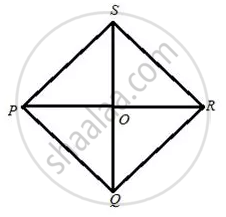# The Perimeter of a Rhombus is 96 Cm and Obtuse Angle of It is 120°. Find the Lengths of Its Diagonals. - Mathematics

Sum

The perimeter of a rhombus is 96 cm and obtuse angle of it is 120°. Find the lengths of its diagonals.

#### Solution

Since in a rhombus all sides are equal.

The diagram is shown below:Therefore PQ = (96)/(4) = 24 cm, Let ∠ PQR = 120°.

We also know that in rhombus diagonals bisect each other perpendicularly and diagonals bisect the angle at vertex.

Hence POR is a right angle triangle and

POR = (1)/(2) ("PQR") = 60°

sin 60° = "Perp."/"Hypot." = "PO"/"PQ" = "PO"/(24)
But
sin 60° = sqrt(3)/(2)

"PO"/(24) = sqrt(3)/(2)

PO = 12sqrt(3) = 20.784

Therefore,

PR = 2PO
= 2 x 20.784
= 41.568 cm

Also,
cos 60° = "Base"/"Hypot" = "OQ"/(24)
But
cos 60° = (1)/(2)

"OQ"/(24) = (1)/(2)

OQ = 12

Therefore, SQ = 2 x OQ

= 2 x 12

= 24 cm
So, the length of the diagonal PR = 41.568 cm and SQ = 24 cm.

Concept: Solution of Right Triangles
Is there an error in this question or solution?

#### APPEARS IN

Selina Concise Mathematics Class 9 ICSE
Chapter 24 Solution of Right Triangles [Simple 2-D Problems Involving One Right-angled Triangle]
Exercise 24 | Q 20 | Page 304